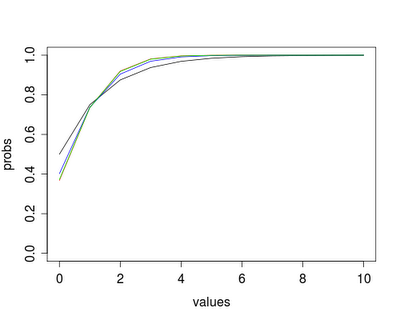## Tuesday, March 15, 2011

### Example 8.30: Compare Poisson and negative binomial count modelsHow similar can a negative binomial distribution get to a Poisson distribution?

When confronted with modeling count data, our first instinct is to use Poisson regression. But in practice, count data is often overdispersed. We can fit the overdispersion in the Poisson (Section 4.1) using quasi-likelihood methods, but a better alternative might be to use a negative binomial regression (section 4.1.5). Nick has a paper exploring these models (and others) in an application.

One concern about this is how well the negative binomial might approximate the Poisson, if in fact a Poisson obtains.

We present here a function and a macro to explore how similar the negative binomial can get to the Poisson, if we keep the means of the distributions equal. But before doing so, it will be helpful to review their definitions:

The Poisson is defined as P(Y=y | l) = [e^(-l)l^y]/y!
and the negative binomial as: P(X=x | n,p) = [(n + x + 1)! / (x!)(n+1)!] p^n (1-p)^x

In the Poisson, the mean is l, while the negative binomial counts the number of failures x before n successes, where the probability of success is p. The mean of X is np/(1-p). There are several characterizations of the negative binomial.

R

In R, the pnbinom() function (section 1.10) can be called either with the parameters n and p given above, or by specifying the mean mu and a dispersion parameter (denoted size), where mu = np/(1-p) as above. It's convenient to parameterize via the mean, to keep the negative binomial mean equal to the Poisson mean.

Our function will accept a series of integers and a mean value as input, and plot the Poisson cumulative probabilities and the negative binomial cumulative probabilities for three values of n. We make use of the type="n" option in the plot() function (section 5.1.1) and add the negative binomial values with the lines() function (section 5.2.1).
`poissonvsnb = function(values,mean)  {  probs = ppois(values,mean)  plot(y=probs, x=values, type="n", ylim=c(0,1))  lines(y=probs, x=values, col="red")  readline("Poisson shown. Press Enter to continue...")  nbprobs1 = pnbinom(values, mu=mean, size=1)  nbprobs5 = pnbinom(values, mu=mean, size=5)  nbprobs40 = pnbinom(values, mu=mean, size=40)  lines(y=nbprobs1, x=values, col="black")  lines(y=nbprobs5, x=values, col="blue")  lines(y=nbprobs40, x=values, col="green")}poissonvsnb(0:10,1)`

The result is shown above. The red line representing the Poisson is completely overplotted by the negative binomial with size=40. This can be seen when running live, due to the readline() statement, which waits for input before continuing.

SAS

In SAS, the cdf function (section 1.10) does not have the flexibility of parameterizing directly via the mean. To add to the confusion, SAS uses another characterization of the negative binomial, which counts the number of successes x before n failures with the effect that the mean is now n(1-p)/p. Thus is we want to hold the mean constant, we need to solve for p and find probabilities from the distribution where p = n/(n + mu).

To make this process a little less cumbersome to type, we'll also demonstrate the use of proc fcmp, which allows you to compile functions that can be used in data steps and some other procedures. In general, it works as you might hope, with a function statement and a return statement. The only hassle is telling SAS where to store the functions and where to find them when they're needed.

`proc fcmp outlib=sasuser.funcs.test;function poismean_nb(mean, size);  return(size/(mean+size));  endsub;run;options cmplib = sasuser.funcs;run;`

Now we're ready to write a macro to replicate the R function. Note how the new function is nested within the call to the cdf function, with the appropriate size parameter. The overlay option allows plotting several y values on the same x axis; the r option to the symbol statement (section 5.1.19) keeps the symbol in effect for several y values. SAS generates a legend easily; this allows us to see the (mostly overplotted) Poisson. Using readline() to pause the output (as in R) is not available.

As a suggestion about how to write macros in SAS, I left this one a little messy. I first wrote the code to make the plot once, with the number of X values and the mean specified in the code with fixed values. This makes two extra lines of code, but when I converted to a macro, I only needed to change the fixed values to the macro parameters. For elegance, I would omit the first two lines and replace the later occurrences of n and mean with the macro parameters.
`%macro nbptest(maxn, mean);data nbp;n = &maxn;mean = &mean;do i = 0 to n;  probpois = cdf("POISSON", i, mean);  probnb1 = CDF("NEGBINOMIAL", i, poismean_nb(mean, 1), 1);  probnb5 = CDF("NEGBINOMIAL", i, poismean_nb(mean, 5), 5);  probnb40 = CDF("NEGBINOMIAL", i, poismean_nb(mean, 40), 40);  output;  end;run;axis1 order = (0 to 1 by .2) minor=none ;symbol1 v=none i=j r=4;proc gplot data=nbp;plot (probpois probnb1 probnb5 probnb40)*i /        overlay vaxis=axis1 legend;run; quit;%mend;%nbptest(10,2);`

The results are shown below. The negative binomial approaches the Poisson very closely as size increases, holding the mean constant.#### 1 comment:

Anonymous said...

proc genmod can also be used for the Pascal loi (Negative binomial)